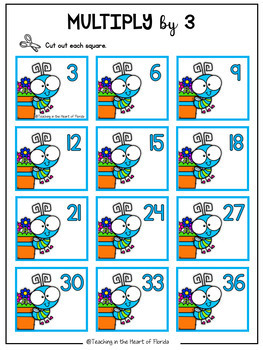# Multiplication Math Centers | Multiplication Practice | Skip-Counting Activity

Rated 4 out of 5, based on 1 reviews
1 Rating;
3rd - 4th, Homeschool
Subjects
Resource Type
Standards
Formats Included
• PDF
Pages
22 pages
Report this resource to TPT

### Description

Your 3rd-grade students will love this fun and engaging multiplication skip counting activity that will help them practice multiplication facts 2 through 12! Perfect for math center activities, this resource can be used all year long to help students of all math levels practice this important math fact strategy. This multiplication skip counting math activity covers math facts from 2's to 12's which makes it easy to use for differentiation.

What I love about this activity is that so easy for you - just print and laminate - and you will use it for years!

⭐ PLEASE SEE THE PREVIEW FOR A CLOSER LOOK!

──────────────────────────────────

Included in this multiplication skip counting math resource:

• 11 Multiplication Work Mats for Facts 2 -12
• Multiplication Cards for Facts 2-12

──────────────────────────────────

HOW TO USE THIS MULTIPLICATION SKIP COUNTING RESOURCE IN YOUR CLASSROOM:

• Small Group Math Instruction
• Math Centers
• Partner Work
• Bellwork
• Early and Fast Finishers

──────────────────────────────────

Math Standards Covered in This Resource:

3.OA.A.1 - Interpret products of whole numbers, e.g., interpret 5 × 7 as the total number of objects in 5 groups of 7 objects each.

3.OA.c.7 - Fluently multiply and divide within 100, using strategies such as the relationship between multiplication and division (e.g., knowing that 8 × 5 = 40, one knows 40 ÷ 5 = 8) or properties of operations. By the end of Grade 3, know from memory all products of two one-digit numbers.

──────────────────────────────────

──────────────────────────────────

"Don't forget to leave feedback -you will receive TPT credits that you can use toward future purchases!

Thank you for visiting Teaching in the Heart of Florida!

Total Pages
22 pages
Included
Teaching Duration
1 Year
Report this resource to TPT
Reported resources will be reviewed by our team. Report this resource to let us know if this resource violates TPT’s content guidelines.

### Standards

to see state-specific standards (only available in the US).
Interpret products of whole numbers, e.g., interpret 5 × 7 as the total number of objects in 5 groups of 7 objects each. For example, describe a context in which a total number of objects can be expressed as 5 × 7.
Fluently multiply and divide within 100, using strategies such as the relationship between multiplication and division (e.g., knowing that 8 × 5 = 40, one knows 40 ÷ 5 = 8) or properties of operations. By the end of Grade 3, know from memory all products of two one-digit numbers.